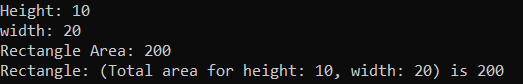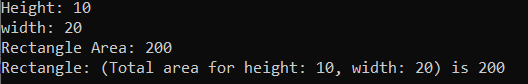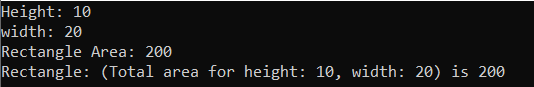## ReadOnly Structs in C# with Examples

In this article, I am going to discuss a new feature introduced with C# 8.0 i.e. Readonly Structs in C# with Examples. Now it is possible to declare the members of a struct as read-only. C# 8.0 is supported on .NET Standard 2.1, .NET Core 3.1, and any higher versions of .NET Core Framework. So, in this article, I am going to use Visual Studio 2022 and .NET 6 to Create a .NET 6 Console Application to demonstrate the need and use of Readonly Struct in C# with Examples.

In C#, we can declare a field using the readonly modifier. The readonly modifier indicates that the assignment to the fields is only part of the declaration or in a constructor of the same class. The readonly fields in C# can only be assigned or reassigned multiple times only at the declaration or in a constructor. They are not assigned after the constructor’s exit. If the readonly modifier is used with a value type field, then the field is immutable. And if the readonly modifier is used with a reference type field, then the readonly modifier prevents the field from being replaced by the different instances of the reference type.

The readonly keyword is a C# modifier that is used to limit access to all the data members of a struct. If the readonly modifier is used in the declaration of a struct, then:

1. The members of the struct are read-only.
2. None of the members can have setters i.e. they only have getters.
3. A parameterized constructor must be used to initialize the data members of a struct.
4. The struct is immutable.
5. The “this” variable cannot be changed in any other method except the constructor. That means the struct readonly members can only be changed through the constructor.

If you do not want to declare the whole structure type as read-only, then you can apply the readonly modifier to the structure member. When we apply the struct member as readonly, then those members don’t modify the state. It is more granular than applying the readonly modifier to a struct declaration.

##### Example to Understand Structure in C#:

Let us first understand the Structure in C#. Please have a look at the below example. In the below example, the structure Rectangle has two properties Height and Width. It calculates the area in another field called Area. And finally, we override the ToString() method which will return a string that contains information about the rectangle object.

```using System;
{
//Creating a Structure using the struct
//struct in C# are value type
public struct Rectangle
{
public double Height { get; set; }
public double Width { get; set; }

//Property Expression Bodied Member
public double Area => (Height * Width);

//Constructor Initializing the Height and Width Properties
public Rectangle(double height, double width)
{
Height = height;
Width = width;
}

//Overriding the Object Class ToString() Method to return the Height, Width, and Area
//But ToString Method is not chaning the Values of Height, Width, and Area Properties
public override string ToString()
{
return \$"(Total area for height: {Height}, width: {Width}) is {Area}";
}
}

class Program
{
static void Main(string[] args)
{
//Creating an Instance of the Rectangle Class
Rectangle rectangle = new Rectangle(10, 20);

//Get the Height of Rectangle
Console.WriteLine("Height: " + rectangle.Height);

//Get the Width of Rectangle
Console.WriteLine("width: " + rectangle.Width);

//Get the Area of Rectangle
Console.WriteLine("Rectangle Area: " + rectangle.Area);

//Get the Height, Width, and Area of Rectangle
//In this case, it will internally invoke the override ToString Method
Console.WriteLine("Rectangle: " + rectangle);
}
}
}
```
###### Output:##### Readonly Member of a Structure in C#:

As you can see in the previous example, the ToString() method doesn’t modify the state of the structure, so we can add the readonly modifier to the declaration of the ToString() method as shown in the below example.

```using System;
{
//Creating a Structure using the struct
//struct in C# are value type
public struct Rectangle
{
public double Height { get; set; }
public double Width { get; set; }

//Property Expression Bodied Member
public double Area => (Height * Width);

//Constructor Initializing the Height and Width Properties
public Rectangle(double height, double width)
{
Height = height;
Width = width;
}

//Overriding the Object Class ToString() Method to return the Height, Width, and Area
//But ToString Method is not chaning the Values of Height, Width, and Area Properties
{
return \$"(Total area for height: {Height}, width: {Width}) is {Area}";
}
}

class Program
{
static void Main(string[] args)
{
//Creating an Instance of the Rectangle Class
Rectangle rectangle = new Rectangle(10, 20);

//Get the Height of Rectangle
Console.WriteLine("Height: " + rectangle.Height);

//Get the Width of Rectangle
Console.WriteLine("width: " + rectangle.Width);

//Get the Area of Rectangle
Console.WriteLine("Rectangle Area: " + rectangle.Area);

//Get the Height, Width, and Area of Rectangle
//In this case, it will internally invoke the override ToString Method
Console.WriteLine("Rectangle: " + rectangle);
}
}
}
```

Once you add the readonly modifier to the ToString() method, then you will not get any error, rather you will see the following warning.

Call to Non-Readonly member ‘Rectangle.Area.get’ from a ‘readonly’ member results in an implicit copy of ‘this’

The compiler warns you when it needs to create an implicit copy of this. The Area property doesn’t change state, so we can fix this warning by adding the readonly modifier to the declaration of the Area property as shown in the below code.

```using System;
{
//Creating a Structure using the struct
//struct in C# are value type
public struct Rectangle
{
public double Height { get; set; }
public double Width { get; set; }

//Property Expression Bodied Member
public readonly double Area => (Height * Width);

//Constructor Initializing the Height and Width Properties
public Rectangle(double height, double width)
{
Height = height;
Width = width;
}

//Overriding the Object Class ToString() Method to return the Height, Width, and Area
//But ToString Method is not chaning the Values of Height, Width, and Area Properties
{
return \$"(Total area for height: {Height}, width: {Width}) is {Area}";
}
}

class Program
{
static void Main(string[] args)
{
//Creating an Instance of the Rectangle Class
Rectangle rectangle = new Rectangle(10, 20);

//Get the Height of Rectangle
Console.WriteLine("Height: " + rectangle.Height);

//Get the Width of Rectangle
Console.WriteLine("width: " + rectangle.Width);

//Get the Area of Rectangle
Console.WriteLine("Rectangle Area: " + rectangle.Area);

//Get the Height, Width, and Area of Rectangle
//In this case, it will internally invoke the override ToString Method
Console.WriteLine("Rectangle: " + rectangle);
}
}
}
```

Now, you will not get any warning. Run the application and you should get the output as expected as shown in the below image.Note: The readonly modifier is necessary on a read-only property. The compiler doesn’t assume get accessors don’t modify state, you must declare readonly explicitly. The Auto-implemented properties are an exception, the compiler will treat all auto-implemented getters as readonly, so in the above example, there is no need to add the readonly modifier to the Height and Width properties.

In readonly structure, we declare the structure with the readonly modifier and readonly structure indicates that the given structure is immutable. When you create a readonly structure, it is necessary to use a readonly modifier with its fields, if you do not do this, then the compiler will give an error. Even, if you need to use only get accessors, if you use set accessors, then you will get an error.

For a better understanding, please have a look at the below example. In the below example, we declare the structure as readonly as well as the Height and Width fields as readonly with the get accessors only.

```using System;
{
//Creating a Structure using the struct
//struct in C# are value type
{
public readonly double Height { get; }
public readonly double Width { get; }

//Property Expression Bodied Member
public double Area => (Height * Width);

//Constructor Initializing the Height and Width Properties
public Rectangle(double height, double width)
{
Height = height;
Width = width;
}

//Overriding the Object Class ToString() Method to return the Height, Width, and Area
//But ToString Method is not chaning the Values of Height, Width, and Area Properties
public override string ToString()
{
return \$"(Total area for height: {Height}, width: {Width}) is {Area}";
}
}

class Program
{
static void Main(string[] args)
{
//Creating an Instance of the Rectangle Class
Rectangle rectangle = new Rectangle(10, 20);

//Get the Height of Rectangle
Console.WriteLine("Height: " + rectangle.Height);

//Get the Width of Rectangle
Console.WriteLine("width: " + rectangle.Width);

//Get the Area of Rectangle
Console.WriteLine("Rectangle Area: " + rectangle.Area);

//Get the Height, Width, and Area of Rectangle
//In this case, it will internally invoke the override ToString Method
Console.WriteLine("Rectangle: " + rectangle);